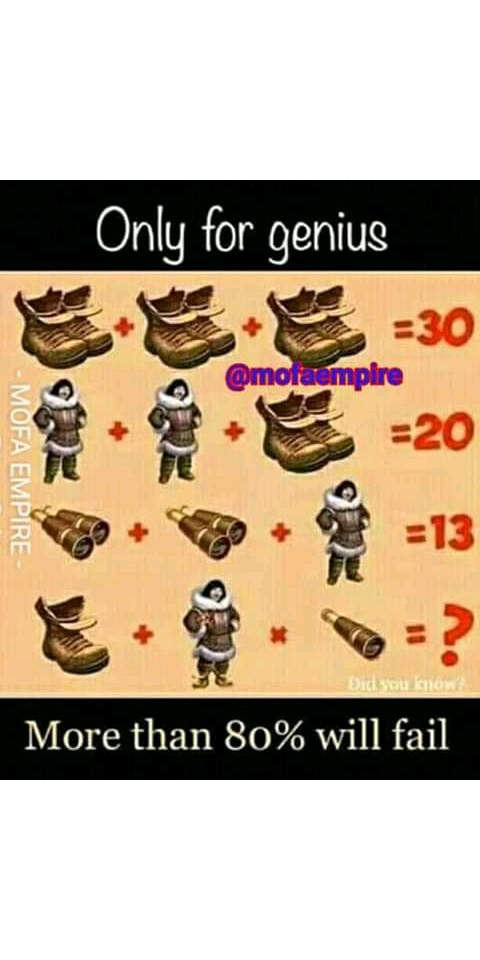# The man, shoes and telescope puzzle answer.

The man, shoes and telescope puzzle answer.This puzzle is very technical, if you don't look and study it well, you will get a right but wrong answer.

When I posted it on Facebook, many people failed it, some got answers like 48, 20 and 24. If this is your first time of seeing the puzzle, solve it and compare with my own bellow.

I will solve each equation to look for the values of the variables, use BODMAS or PEMDAS to solve the final equation.

Solution:
Equation One
A pair of shoes + a pair of shoes + a pair shoes = 30.
Impplies 3 pairs of shoes = 30,
Implies Pair of shoes = 30/3,
Implies Pair of shoes = 10.

If a pair of shoes = 10
then, a shoe = 5.

Equation two:
I will substitute the value of pair of shoes (10) in this equation.
2men + pair of shoes = 20,
implies 2men + 10 = 20.
Implies  2men = 20 - 10
Implies 2men = 10
A man = 10/2
A man = 5.

Equation three:
I will subside the value of man (5) in this equation.
2 pairs of telescope + 5 = 13,
2 pairs of telescope = 13 - 5
2 pairs of telescope = 8,
A pair of telescope = 8/2
Implies Pair of telescope = 4.
If a pair of telescope = 4
then, telescope = 2.

Final equation:
As mentioned earlier, I will use PEMDAS to solve this final equation but before I do so, I will vivitly explain the meaning of PEMDAS.

PEMDAS is a mathematical acronym which stands for:
P= Parentheses ()
E=Exponent
M=Multiplication
D=Division
S=Subtraction. With PEMDAS, to solve algebraic expressions, it is further grouped into four important stages in order of merit.
First: Parenthesis () or Bracket [].
Second: Exponents
Third: multiplication and Division.
This stage is very important to note because this is where the left to right rule, if not applied may give different answers.check example 1 above.

I will rewrite the final equation for you to understand better.
This is where the you will find the trick in the puzzle.

A shoe + A man wearing shoes and holding a pair of telescope × a telescope = ?.

The value of a shoe = 5.
Man = 5.
A pair of telescope = 4
Telescope = 2.
I will subside these values in the equation above.
5 + ( 5+ 10 + 4 ) × 2 =?
With the use of  PEMDAS, I will solve the bracket first.
5+ 10 + 4 = 19.
Implies 5 + 19 × 2 = ?
5 + 38 = ?
43 = ?

Hence, the answer to the puzzle is 43. If you have different views let me know via comment.

Check answer to this trending Fowl, banana and egg Puzzle